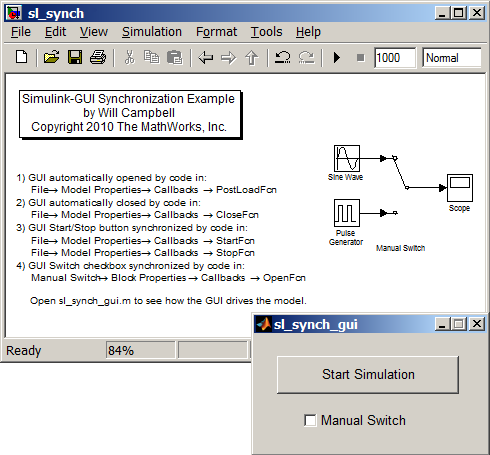# Synchronizing a MATLAB GUI and a Simulink Model

Jiro's pick this week is Simulink GUI Synchronization by our fellow MathWorker Will Campbell.

Here's a little something new. We've been mostly looking at MATLAB entries in this blog, but today we'll take a look at a Simulink example. Even though this is a simple example, I find this very useful and pedagogical. Imagine that you want to build a graphical user interface that controls your Simulink model (You know how I'm all about GUIs!). This is not uncommon and can be accomplished by using functions like set_param and get_param. But what if you want to be able to control the model directly through Simulink as well as through the MATLAB interface? In that case, you want to make sure that the interface accurately reflects the current state of the model.

In this example, Will shows how you can create a MATLAB GUI that not only drives and manipulates your Simulink model, but also stays synchronized to the current state. You too can now drive and manipulate your Simulink model from either environment!